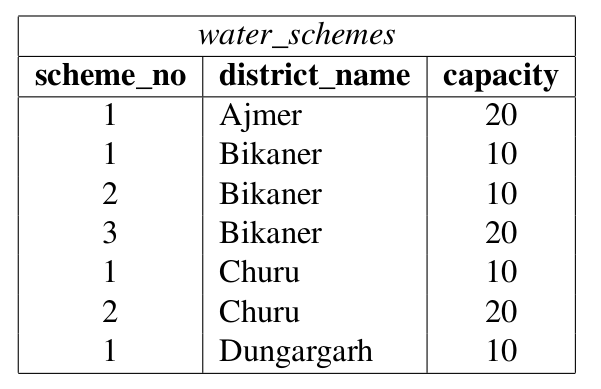Open in App
Not now

# GATE | GATE-CS-2016 (Set 2) | Question 62

• Difficulty Level : Easy
• Last Updated : 28 Jun, 2021

Consider the following database table named water_schemes :The number of tuples returned by the following SQL query is

```with total(name, capacity) as
select district_name, sum(capacity)
from water_schemes
group by district_name
with total_avg(capacity) as
select avg(capacity)
from total
select name
from total, total_avg
where total.capacity >= total_avg.capacity```

(A) 1
(B) 2
(C) 3
(D) 4

Explanation: First group by district name is performed and total capacities obtained as following

```   Ajmer 20
Bikaner 40
Charu 30
Dungargarh 10 ```

Then average capacity is computed,

```Average Capacity = (20 + 40 + 30 + 10)/4
= 100/4
= 25.
```

Finally districts with more than average are selected.

```Bikaner is 40 which is greater than average (25)
Charu is 30 which is also greater than average (25).